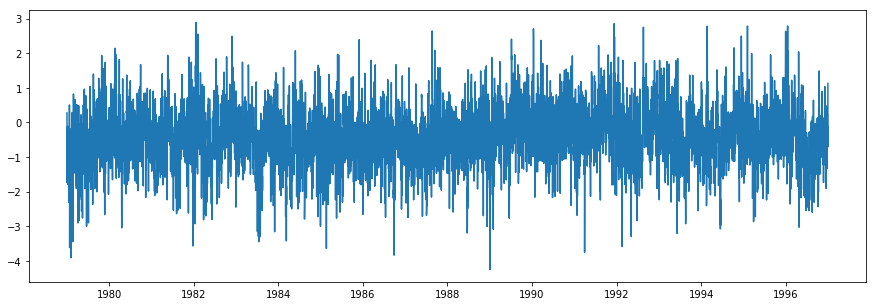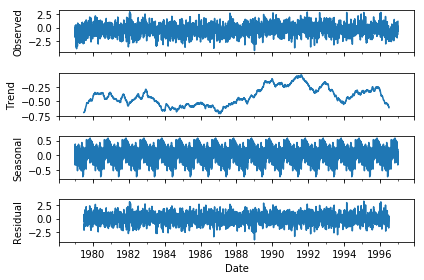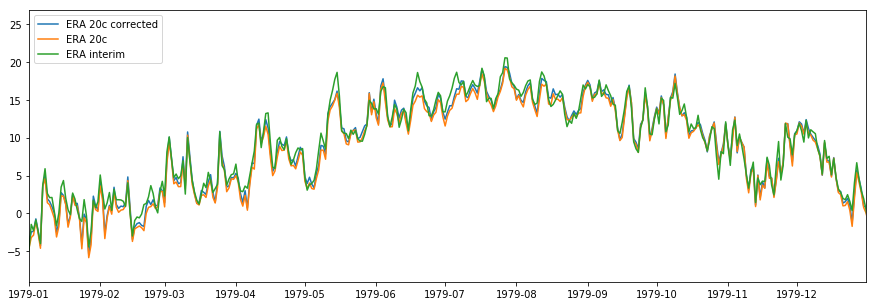# The list of cities¶

The statistics office of the European Union, Eurostat, runs an "Urban Audit" at regular intervals, carring out perceptions surveys in a series of cities across the Union. It is hard to understand precisely which cities are taken into account, as the list varies in length between 70 and 1000, depending on the data series.

We took the longest possible list of cities, so that we'd have the EU code for as many cities as possible (e.g. Narva, in Estonia, is EE003C1). The rationale behind this decision was to enable us, later on in the project, to easily reconcile our cities with any data that Eurostat possesses on cities, such as questions of the Urban Audit survey related to perceptions of climate change.

There is no clear logic in the way the list was constructed ; populations range from 20,000 to 8 million. We assumed that each member state gave Eurostat a list representative of its geography and of its urban diversity, which is what we were after.

Later in the project, 53 cities were added, following requests by local media outlets. These do not have a Eurostat code and are refered by name only.

You can see the complete data and cities list in a Gitlab repository.

## Cleaning the list¶

We started from the population list, which contains many encoding errors, duplicates (e.g. "Amsterdam" and "Greater Amsterdam") and peculiarities (the French listed several city groupings as individual cities, for instance).

The list was cleaned and geolocalized with the following program. Out of the 1049 cities listed by Eurostat, we kept 945.

In [ ]:
import pandas as pd
import re, requests, json, time

# Creates a field with both cities and country
df["Country"] = df["CITIES"].str[0:2]

# Removes countries
df = df.loc[df["CITIES"].str.len() > 2]

# Removes "greater cities", clean names and other non-clean names
df = df.loc[df["CITIES_LABEL"].str.contains("CA") == False] # e.g. CA Nord Essone
df = df.loc[df["CITIES_LABEL"].str.contains("CC") == False] # e.g. CC Val de Loire
df = df.loc[df["CITIES"].str.contains("K1\$", regex=True) == False] # e.g. Glasgow (K1 in the code seems to be for greater cities)
df["CITIES_LABEL"] = df["CITIES_LABEL"].map(lambda x: x[0:x.find("/")].strip() if x.find("/")>0 else x) #e.g. Bruxelles/Brussels
df["CITIES_LABEL"] = df["CITIES_LABEL"].map(lambda x: x[0:x.find(" - ")].strip() if x.find(" - ")>0 else x) #e.g. Lens - Liévin
df["CITIES_LABEL"] = df["CITIES_LABEL"].str.replace('Greater ', '') # e.g. Greater Amsterdam
df["CITIES_LABEL"] = df["CITIES_LABEL"].map(lambda x: re.sub(r'(.+), (.+)',r'\2 \1', x) if x.find(", ")>0 else x) # e.g. Línea de la Concepción, La
df["CITIES_LABEL"] = df["CITIES_LABEL"].str.replace('ĝ', 'ø') # e.g. Tromsĝ
df["CITIES_LABEL"] = df["CITIES_LABEL"].str.replace('', 'ã') # e.g. Guimares
df["CITIES_LABEL"] = df["CITIES_LABEL"].str.replace('ċ', 'å') # e.g. Västerċs
df["CITIES_LABEL"] = df["CITIES_LABEL"].str.replace('ġ', 'ő') # e.g. Gyġr
df["CITIES_LABEL"] = df["CITIES_LABEL"].str.replace('Ċ', 'Aa') # e.g. Ċrhus
df["CITIES_LABEL"] = df["CITIES_LABEL"].str.replace('Athina', 'Athens')
df["CITIES_LABEL"] = df["CITIES_LABEL"].str.replace('Halle an der Saale', 'Halle (Saale)')

# Some British cities still are unclean ; would need manual cleaning after verification of city status
# (e.g is it a conurbation or a real city?)

# The following steps let us keep only the rows where a population figure is available,
# and keeps only the most recent

# Removes all years with no population
df = df.loc[df["Value"].str.contains(":") == False]
# Removes duplicates
df = df.drop_duplicates(subset="CITIES_LABEL", keep='last')

# Takes first lines only for test purposes
latitudes = []
longitudes = []

for index, item in df.iterrows():
if item["Country"] == "EL":
country = "GR"
elif item["Country"] == "UK":
country = "GB"
else:
country = item["Country"]

time.sleep(2)

url = "http://nominatim.openstreetmap.org/search/?format=json&q=%s&countrycodes=%s&limit=1" % (item["CITIES_LABEL"], country)
r = requests.get(url)
if data != []:
latitudes.append(data["lat"])
longitudes.append(data["lon"])
print(data["display_name"])
else:
latitudes.append("")
longitudes.append("")
print("Fail for " + item["CITIES_LABEL"])

df["Latitude"] = [x for x in latitudes]
df["Longitudes"] = [x for x in longitudes]

df.to_csv("../Data/cities.csv", sep='\t', encoding='utf-8')


# Getting the data from ECMWF¶

After a telephone chat with climate scientists at ECMWF, we proceeded with gathering historical temperature and snow cover data on each city in the list.

The code is based on a file provided by ECMWF on their website. For each month between January 1900 and December 2017, it queries the ECMWF database and receives the temperature (in degrees Kelvin) and the snow cover (in meters) for the coordinates of each city at midnight, 6 am, 12 am and 6 pm.

The resulting data is then averaged per day, converted to degrees Celsius and centimeters and zipped. The program (written for Python2.7) ran on an Ubuntu server rented from Amazon Web Services during two weeks.

In [ ]:
import os, sys, csv, math, subprocess
import pandas as pd
import numpy as np
sys.path.append('/home/ubuntu/eccodes/lib/python2.7/site-packages/eccodes')
sys.path.append('/home/ubuntu/eccodes/lib/python2.7/site-packages/gribapi')

from ecmwfapi import ECMWFDataServer
from eccodes import *

def get_met_data(date, param, area):
fname = date + '_' + param + '_' + area + '.grib'
fname = fname.replace('/', '-')
fname = fname.replace('\\', '-')
target = os.path.join(met_data_dir, fname)
server = ECMWFDataServer()
if os.path.exists(target):
print "\nUsing existing meteorological data file %s \n" % target
else:
server.retrieve({
"class" : class_ec,
"dataset": dataset,
"date" : date,
"stream" : "oper",
"expver" : "1",
"levtype" : "sfc",
"param" : param,
"grid" : "0.75/0.75", #setting grid to ll or F128 causes interpolation but is required for area.
"area" : area,
"time" : "00:00:00/06:00:00/12:00:00/18:00:00",
"type" : "an",
"target" : target,
})
return target

param = "141.128/167.128"
met_data_dir = 'data/tmp'
in_loc = 'cities_list.csv'
lid = 'city_name'
llat = 'lat'
llon = 'lon'

for year in range(1979, 2018):
for month in range(1,13):
if not (year == 1979 and month == 1):
print "Now doing month %s of year %s" % (month, year)
year = str(year)
if month < 10:
month = "0"+ str(month)
else:
month = str(month)
dataset = "interim"

if dataset == "era20c":
class_ec = "e2"
else:
class_ec = "ei"

if month == "02":
max_days = "28"
elif month in ["01", "03", "05", "07", "08", "10", "12"]:
max_days = "31"
else:
max_days = "30"

# Output file:
# If this file already exists it will be overwritten.
out_loc = 'data/%s-%s-%s.csv' % (year, month, dataset)

date_from = "%s-%s-01" % (year, month)
date_to = "%s-%s-%s" % (year, month, max_days)
date = "%s/to/%s" % (date_from, date_to)

# load locations list into pandas dataframe
# get extent of locations list, plus 5 degrees either side as long as we are within 90/-180/-90/180
area = [min(ldf[llat].max()+5,90), max(ldf[llon].min()-5,-180), max(ldf[llat].min()-5,-90), min(ldf[llon].max()+5,180)]
# make the area list into a string to use it in the met_data_file function
area = "/".join(map(str, area))

# make directory for meteorological data
if not os.path.exists(met_data_dir):
os.makedirs(met_data_dir)
# get meteorological data
met_data_file = get_met_data(date, param, area)
m = open(met_data_file)

# get list of grib messages from met data file
mcount = codes_count_in_file(m)
gid_list = [codes_grib_new_from_file(m) for i in range(mcount)]

m.close()

# create output list
o = csv.writer(open(out_loc, 'wb'), delimiter=',')

# write a header line to output file
o.writerow([lid,llat,llon,'Date','Time','Parameter Name', 'Short Name', 'Distance to MetPoint', 'Latitude of MetPoint', 'Longitude of MetPoint', 'Value at MetPoint'])

# iterate over grib messages
for gid in gid_list:

#iterate over location list
for index, row in ldf.iterrows():

# for current location, get nearest point in current grib message
nearest = codes_grib_find_nearest(gid, row[llat], row[llon], npoints=1)
# format nicely
result = [row[lid], round(row[llat],4), round(row[llon],4), codes_get(gid, 'date'), codes_get(gid, 'time'), codes_get(gid, 'name'), codes_get(gid, 'shortName'), round(nearest.distance,4), round(nearest.lat,4), round(nearest.lon,4), nearest.value]

# write result for this grib message / this location to output file
o.writerow(result)

#dispose of grib message
codes_release(gid)

# Splits the files by city and zips them
file_name = '%s-%s-%s.csv' % (year, month, dataset)

for index, row in cities.iterrows():
city_name = row["city_name"]
city_code = row["city_code"]
df_city = df.loc[(df["city_name"] == city_name)]
directory = "data/%s" % city_code
file_name_city = directory + "/" + file_name

# Removes any null or na values in the column "Value at metpoint"
df_city = df_city[pd.notnull(df_city['Value at MetPoint'])]

# Converts date col to datetime
df_city["Date"] = df_city["Date"].astype(int)
df_city["Date"] = df_city["Date"].astype(basestring)
df_city["Date"] = pd.to_datetime(df_city["Date"], format='%Y%m%d', errors='ignore')

# Aggregates per day and per city
table = pd.pivot_table(df_city, values='Value at MetPoint', index=['Date'], columns=['Parameter Name'], aggfunc=np.mean)

# Convert to Celsius
table["2 metre temperature"] -= 273.15

# Convert to cm
table["Snow depth"] *= 100

table.to_csv(directory + "/" + file_name, sep=',', encoding='utf-8')

# Creates a zip for the month and the city
bashCommand = "zip -j %s.zip %s" % (file_name_city, file_name_city)
process = subprocess.Popen(bashCommand.split(), stdout=subprocess.PIPE)
output, error = process.communicate()
# Removes the csv file of the city
bashCommand = "rm %s" % (file_name_city)
process = subprocess.Popen(bashCommand.split(), stdout=subprocess.PIPE)
output, error = process.communicate()
# Removes the csv file with all cities
bashCommand = "rm %s" % (out_loc)
process = subprocess.Popen(bashCommand.split(), stdout=subprocess.PIPE)
output, error = process.communicate()
# Removes the grib file
bashCommand = "rm %s" % (met_data_file)
process = subprocess.Popen(bashCommand.split(), stdout=subprocess.PIPE)
output, error = process.communicate()


# Deduplicating cities¶

ECMWF provides weather data in squares of 80 by 80 kilometers. It analyzes millions of observations (data from weather stations, satellites etc.) for a zone, then averages the values for the square. Such weather data does not take into account micro-climates within the 80x80-kilometer square. This is bad for weather forecasting over a few days, but very good for long-term analyses, because micro-climates, especially in cities, change over time (because cities all grew over the last 117 years).

For each square, we only kept the largest city (which is why Düsseldorf and Bonn did not make it to the final list of cities, only Cologne did) with the following program. 440 cities were discarded ; the final list comprises 505 cities.

In :
import pandas as pd
import numpy as np
import matplotlib.pyplot as plt
import datetime as dt
import random

cities_df["population"] = cities_df["population"].str.replace(" ", "")
cities_df["population"] = pd.to_numeric(cities_df["population"])

cities_df.sort_values(by="population", ascending=False, inplace=True)

cities_df.drop_duplicates(subset=['metpoint_lat_era20c', 'metpoint_lon_era20c'], inplace=True)

cities_df.to_csv("../Data/cities_list_deduplicated.csv", sep=',', encoding='utf-8')


# Merging the ECMWF data sets¶

The ECMWF data is actually two different data sets. One is ERA-20c, which goes from 1900 to 2010, the other is ERA-interim, from 1979 to today. ERA-interim is of much higher quality (there's not much satellite data to process in the early 20th century) and higher resolution (the squares for ERA-20c are 125x125 kilometers large).

We had to slightly modify the data from the 20c data set (1900 to 1979) so that it can be compared with the data from the interim data set (1979 to 2017).

To do so, we compared the data for the period 1979-1997 and looked at the difference in temperature between the two with the following program.

In :
import pandas as pd
import numpy as np
import matplotlib.pyplot as plt
import datetime as dt
import random
from statsmodels.tsa.seasonal import seasonal_decompose

from_year = 1979
to_year = 1997

test_city = random.choice(cities_df["city_code"])

for city, row in cities_df.iterrows():
city_code = row["city_code"]
if city_code == test_city:
city_name = row["city_name"]
print(city_name)
city_era20c = "../Data/data/%s/1900-1997-era20c.csv" % city_code
city_interim = "../Data/data/%s/1979-2018-interim.csv" % city_code

city_era20c_df = pd.read_csv(city_era20c, index_col="Date", parse_dates=True).drop(["Unnamed: 0", "Snow depth"], axis=1)
city_interim_df = pd.read_csv(city_interim, index_col="Date", parse_dates=True).drop(["Unnamed: 0", "Snow depth"], axis=1)

city_era20c_df = city_era20c_df.loc[city_era20c_df.index.year >= from_year]
city_interim_df = city_interim_df.loc[city_interim_df.index.year < to_year]

city_diff = city_era20c_df - city_interim_df

# The following two lines are needed because I forgot to take into account Feb 29s before
city_diff = city_diff.resample('D').mean()
city_diff = city_diff.interpolate()

fig, ax = plt.subplots(1, 1, figsize=(15, 5))
ax.plot(city_diff.index, city_diff["2 metre temperature"])

IslingtonThe difference in temperature before the two data sets is not random, it follows a seasonal pattern. This makes sense, because the squares being averaged to produce this data are not equal. The square under observation in ERA-20c might be a bit more to the South or West than the square of ERA-interim, which explains the seasonal patterns.

To account for these, we decomposed the series above to extract its seasonal component with the following code:

In :
result = seasonal_decompose(city_diff, model='additive', freq=365)
result.plot()
plt.show()We then corrected the original data by removing the seasonal component from it as well as removing the average of the trend with the following code:

In :
era20c_corrected = city_era20c_df - result.seasonal - result.trend.mean()
fig, ax = plt.subplots(1, 1, figsize=(15, 5))
ax.set_xlim([dt.datetime(1979, 1, 1, 0, 0), dt.datetime(1979, 12, 31, 0, 0)])
ax.plot(era20c_corrected.index, era20c_corrected["2 metre temperature"], label="ERA 20c corrected")
ax.plot(city_era20c_df.index, city_era20c_df["2 metre temperature"], label="ERA 20c")
ax.plot(city_interim_df.index, city_interim_df["2 metre temperature"], label="ERA interim")
ax.legend(loc='upper left')

Out:
<matplotlib.legend.Legend at 0x7f43406b2358>The correction was then applied to the data from the 20c data set from 1900 to 1979.

We did run the same program to reconcile the values for snow cover for the two data sets. However, the differences between the two were too large, even after a correction. The data for a city would show a very snowy winter in the 20c data set, for instance, while the interim data set would have recorded only a few days of snow, and vice versa in another year. We subsequently decided to drop the "snow cover" column from our analysis.

At this stage, we had a single data set of temperature averages by day from 1900 to 2017 for each 505 cities.

In [ ]: